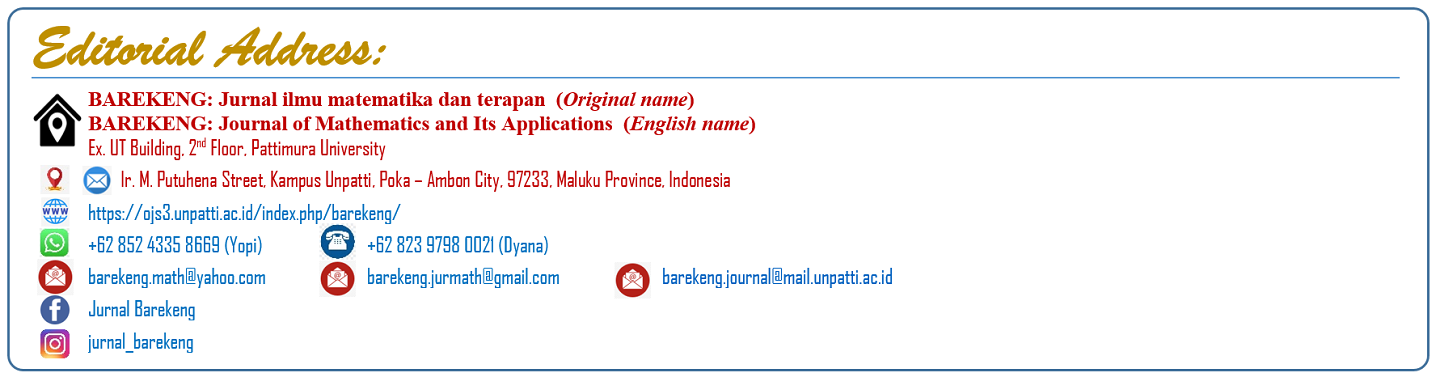# Aim and Scope

BAREKENG: Jurnal Ilmu Matematika dan Terapan is one of the scientific publication media, which publish articles from research papers, literature studies, analysis, and problem solving in Mathematics, about:
- Pure Mathematics (Analysis, Algebra & Number Theory),
- Applied Mathematics (Fuzzy, Artificial Neural Network, Mathematics Modelling & Simulation, etc),
- Control & Optimization,
- Combinatorics
- Ethno-Mathematics,
- Statistics,
- Actuarial Science,
- Logics Mathematics,
- Geometry & Topology,
- Numerical Analysis,
- Mathematic Computation,
- Physic Mathematics
- Mathematics Education.
It cordially contributions from the researcher, lecturer, and teacher of related disciplines. The language used in this journal is English.